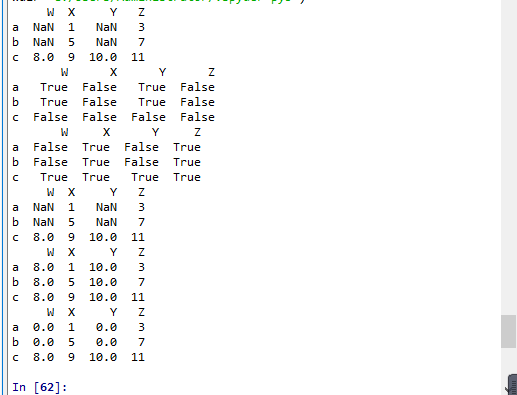# Python 中pandas索引切片讀取數據缺失數據處理問題

更新時間：2019年10月09日 11:11:25   作者：一知....半解我要評論
pandas是一個Python軟件包，提供快速，靈活和富于表現力的數據結構，旨在使使用“關系”或“標記”數據既簡單又直觀。這篇文章主要介紹了pandas索引切片讀取數據缺失數據處理,需要的朋友可以參考下

numpy已經能夠幫助我們處理數據，能夠結合matplotlib解決我們數據分析的問題，那么pandas學習的目的在什么地方呢？ numpy能夠幫我們處理處理數值型數據，但是這還不夠 很多時候，我們的數據除了數值之外，還有字符串，還有時間序列等 比如：我們通過爬蟲獲取到了存儲在數據庫中的數據 比如：之前youtube的例子中除了數值之外還有國家的信息，視頻的分類(tag)信息，標題信息等 所以，numpy能夠幫助我們處理數值，但是pandas除了處理數值之外(基于numpy)，還能夠幫助我們處理其他類型的數據。

pandas是一個Python軟件包，提供快速，靈活和富于表現力的數據結構，旨在使使用“關系”或“標記”數據既簡單又直觀。它旨在成為在Python中進行實際，真實世界數據分析的基本高級構建塊。此外，其更廣泛的目標是成為任何語言中可用的最強大，最靈活的開源數據分析/操作工具。它已經朝著這個目標邁進了。

pandas的常用數據類型

1、Series 一維，帶標簽數組

2、DataFrame 二維，Series容器

(1)Series創建

`pandas.Series(data=None, index=None, dtype=None, name=None, copy=False, fastpath=False)`

data:類數組，可迭代，字典或標量值,包含存儲在系列中的數據。在0.23.0版中進行了更改：如果data是dict，則將為Python 3.6及更高版本維護參數順序。

index：類數組或索引（1d）值必須是可散列的，并且與data的長度相同。允許使用非唯一索引值。如果未提供，則默認為RangeIndex（0，1，2，…，n）。如果同時使用了字典和索引序列，則索引將覆蓋在字典中找到的鍵。

dtype：STR，numpy.dtype，或ExtensionDtype，可選

copy：bool，默認為False,copy輸入數據。

```import pandas as pd
import numpy as np
t = pd.Series(np.arange(12),index= list("asdfghjklpoi"))
print(t)
print(type(t))``````c = {"name":"lishuntao","age":18,"gender":"boy"}
t1 = pd.Series(c)
print(t1)
print(type(t1))
print(t1["name"])
print(t1["gender"])```

```import numpy as np
import pandas as pd

a = {"a":12,"name":"lishuntao","c":"xiaoc","age":18,"gender":"man"}

t1 = pd.Series(a)
print(t1)
print(type(t1))
t2 = pd.Series(a,index=list("abcdf"))
print(t2)```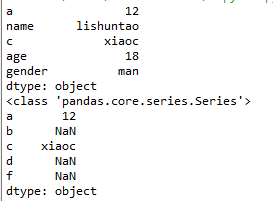numpy中的nan為float，pandas會自動根據數據類型更改series的dtype類型。

Series切片和索引

```import numpy as np
import pandas as pd

a = {"a":12,"name":"lishuntao","c":"xiaoc","age":18,"gender":"man"}

t1 = pd.Series(a)
print(t1)
print(t1[:2])
print(t1)
print(t1[["a","c","gender"]])
print(t1[0:5:2])```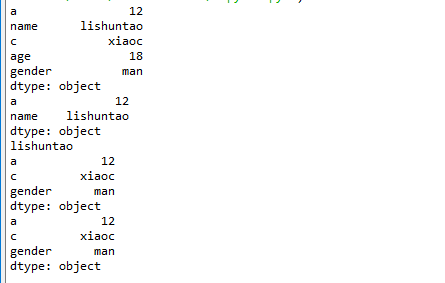```import numpy as np
import pandas as pd

a = np.arange(12)
t1 = pd.Series(a)
print(t1)
print(t1[t1>9])```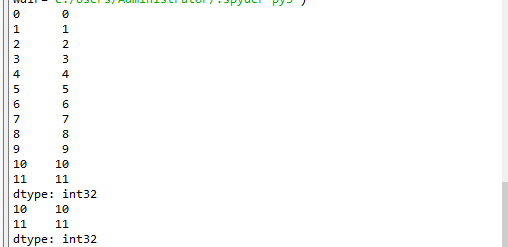Series的索引和值

```import numpy as np
import pandas as pd

a = np.arange(12)
t1 = pd.Series(a)
#print(t1)
print(t1.index)
print(t1.values)```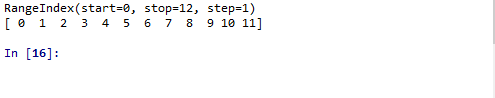```import numpy as np
import pandas as pd

a = np.arange(12)
t1 = pd.Series(a)
print(t1)
print(type(t1.index))
print(type(t1.values))```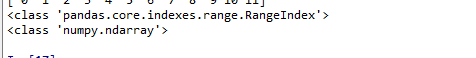Series對象本質上有兩個數組構成，一個數組構成對象的鍵（index，索引），一個數組構成對象的值（values），鍵--->值。

ndarray的很多方法都可以運用與series類型，比如argmax，clip

series具有where方法，但是結果卻不同（下面是官方文檔給出）

`Series.where`(self,cond[,other,inplace,…])Replace values where the condition is False.

```a = np.arange(12)
t1 = pd.Series(a)
print(t1)
#替換條件是False的情況 下面兩個結果一樣
print(t1.where((t1>8),1))
print(pd.Series.where(t1,(t1>4),1))```

pandas之讀取外部數據

現在假設我們有一個組關于狗的名字的統計數據，那么為了觀察這組數據的情況，我們應該怎么做呢？

數據來源：https://www.kaggle.com/new-york-city/nyc-dog-names/data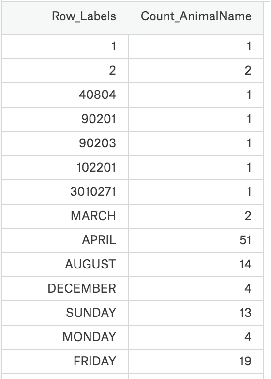```import numpy as np
import pandas as pd
print(t2)
print(type(t2))```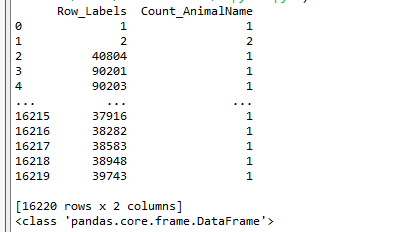`pd.read_sql(sql_sentence,connection)`

(2)DataFrame的創建

`pd.DataFrame（data,index,columns,dtype,copy)`

```import numpy as np
import pandas as pd
t2 = pd.DataFrame(np.arange(12).reshape(3,4))
print(t2)```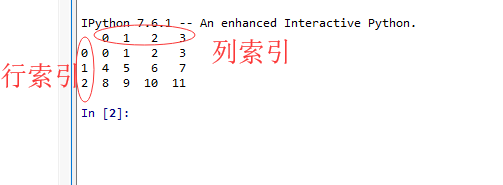```t2 = pd.DataFrame(np.arange(12).reshape(3,4),index=list("abc"),columns=list("WXYZ"))
print(t2)```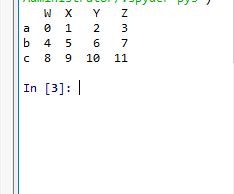DataFrame的基礎屬性

```t2 = pd.DataFrame(np.arange(12).reshape(3,4),index=list("abc"),columns=list("WXYZ"))
print(t2)
print(t2.shape)#顯示行數，列數
print(t2.dtypes)#顯示的是列數據類型
print(t2.ndim)#數據維度2（0,1）
print(t2.index)#行索引
print(t2.columns)#列索引 Index(['W', 'X', 'Y', 'Z'], dtype='object')
print(t2.values)#對象值，二維ndarray的數組```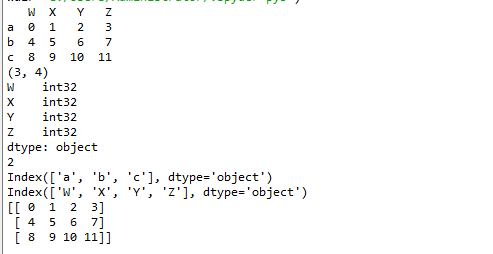DataFrame整體情況查詢

```t2 = pd.DataFrame(np.arange(12).reshape(3,4),index=list("abc"),columns=list("WXYZ"))
print(t2)
print(t2.tail(2))#顯示末尾幾行，默認5行
print(t2.info())#相關信息瀏覽：行數，列數，列索引，列非空值個數，列類型，列類型，內存占用
print(t2.describe())#快速綜合統計結果：計數，均值，標準差，最大值，四分位數，最小值``````pd.DataFrame.sort_values(by="Count_AnimalName",ascending=False)#ascending=True升序排序 by是對那一列排序 輸入列索引鍵
print(t2)

t3 = t2.sort_values(by="Count_AnimalName",ascending=False)
print(t3)```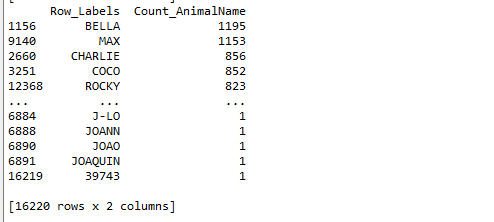pandas之取行或者列

剛剛我們知道了如何給數據按照某一行或者列排序，那么現在我們想單獨研究使用次數前100的數據，應該如何做？

```t2 = pd.read_csv("F:\BaiduNetdiskDownload\youtube_video_data\dogNames2.csv")
print(t2)

t3 = t2.sort_values(by="Count_AnimalName",ascending=False)
print(t3[:100])```pandas之loc取行數據

1、t2.loc 通過標簽索引行數據（標簽）

```print(t2.loc["a","W"])
print(t2.loc["a",["W","Y"]])
print(type(t2.loc["a",["W","Y"]]))
print(t2.loc[["a","b"],["Z","Y"]])
print(t2.loc[:"c",:"Y"])
print(t2.loc["a":"b",["W","Z"]])```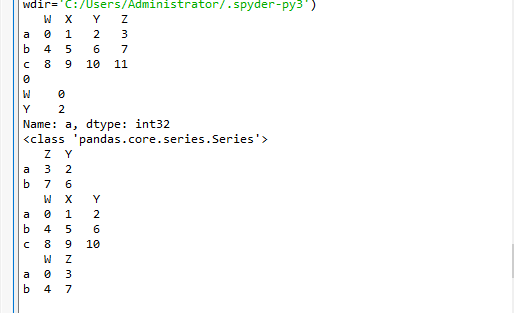2、t2.iloc 通過位置獲取行數據（位置）

```import numpy as np
import pandas as pd

t2 = pd.DataFrame(np.arange(12).reshape(3,4),index=list("abc"),columns=list("WXYZ"))
print(t2)
print(t2.iloc[0:2,0:4])
print(t2.iloc[[0,2],[1,3]])
t2.loc["a","Y"] = 100 #復制操作
print(t2)
t2.iloc[1:2,] = 1000 #復制操作
print(t2)```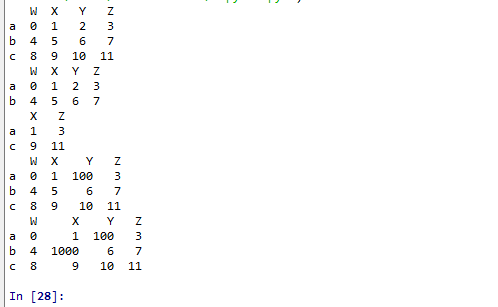pandas之布爾索引（且，或，&，|，）

回到之前狗的名字的問題上，假如我們想找到所有的使用次數超過800的狗的名字，應該怎么選擇？

`print(t2[t2["Count_AnimalName"]>800])`

`print(t2[(t2["Row_Labels"].str.len()>4)&(t2["Count_AnimalName"]>700)])`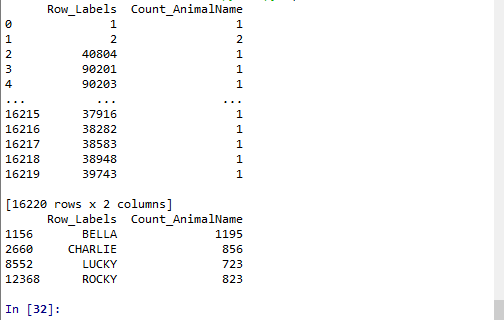pandas之字符串方法觀察這組數據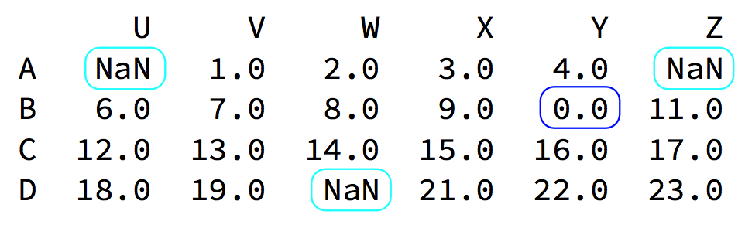我們的數據缺失通常有兩種情況： 一種就是空，None等，在pandas是NaN(和np.nan一樣) 另一種是我們讓其為0（藍色框中）

對于NaN的數據，在numpy中我們是如何處理的？

在pandas中我們處理起來非常容易 判斷數據是否為NaN：pd.isnull(df),pd.notnull(df)

處理方式1：刪除NaN所在的行列

`　dropna (axis=0, how='any', inplace=False)`

處理方式2：填充數據，

`　t.fillna(t.mean()),t.fiallna(t.median()),t.fillna(0)`

處理為0的數據：t[t==0]=np.nan 當然并不是每次為0的數據都需要處理 計算平均值等情況，nan是不參與計算的，但是0會

```import numpy as np
import pandas as pd

t2 = pd.DataFrame(np.arange(12).reshape(3,4),index=list("abc"),columns=list("WXYZ"))
#print(t2)
t2.loc[:"b",["W","Y"]] = np.nan
print(t2)
print(pd.isnull(t2))
print(pd.notnull(t2))
#print(t2.dropna(axis=0,how="all",inplace=False))
#any只要含NaN就刪除前面規定的行列，all需要的是行列全部為NAN才能刪除
#填充數據
#print(t2.fillna(t2.mean()))
print(t2)
print(t2.fillna(t2.median()))
print(t2.fillna(0))```